Problem

# The following table shows a partially completed ANOVA table for a two-factor factorial e...

The following table shows a partially completed ANOVA table for a two-factor factorial experiment: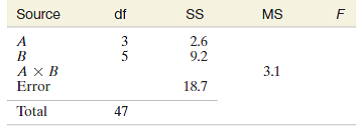a. Complete the ANOVA table.

b. How many levels were used for each factor? How many treatments were used? How many replications were performed?

c. Find the value of the sum of squares for treatments. Test to determine whether the data provide evidence that the treatment means differ. Use α = .05.

d. Is further testing of the nature of factor effects warranted? If so, test to determine whether the factors interact. Use α = .05. Interpret the result.

#### Step-by-Step Solution

Solution 1

A partially completed ANOVA for a two factor factorial experiment is provided in the problem

 Source df SS MS F A 3 2.6 B 5 9.2 A X B 3.1 Error 18.7 Total 47

a. The General ANOVA summary table for a two factorial experiment with r Replications, a levels of Factor A and b levels of Factor B is as below:

 Source df SS MS F A (a-1) SSA MSA = SSA/(a-1) MSA/MSE B (b-1) SSB MSB=SSB/(b-1) MSB/MSE A X B (a-1)(b-1) SSAB MSAB=SSAB/(a-1)(b-1) MSAB/MSE Error ab(r-1) SSE MSE=SSE/ab(r-1) Total (n-1) SS(Total)

Using the above general formulas, the completed ANOVA table is as below:

 Source df SS MS F A 3 2.6 0.9 1.1 B 5 9.2 1.8 2.3 A X B 15 46.5 3.1 3.9 Error 24 18.7 0.8 Total 47 77

Values updated in the table are highlighted in color green.

b. Using the information in the above completed ANOVA table, we answer the following questions.

i. Number of levels of Factor A are 4 and that for Factor B are 6

ii. There were 24 (4 X 6) treatments that were used in the experiment

iii. Two replications were performed for each treatment

c. We obtain the sum of square for treatment

Sum of Square for treatment (SST) = SSA + SSB + SSAB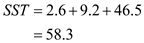Sum of Square for Treatment = 58.3

Degrees of Freedom for treatment = DoF for A + DoF for B + DoF for AB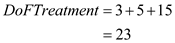The F-ratio for treatments is F= 3.1, which exceeds the tabular value of F0.05= 1.99 for 23 numerator and 24 denominator degrees of freedom. We conclude that at least two treatments have different mean responses.

d. In part c above, we concluded that there is difference in the treatment means for at least two treatments.

So, we proceed to test the interaction effect on the mean responses.

The hypotheses to be tested are as follows:

H0: Factor A and Factor B do not interact to affect the response mean

Ha: Factors A and B do interact to affect the response mean

Test statistic: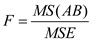Rejection region: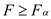, based on (a-1)(b-1) numerator and (n-ab) denominator degrees of freedom.

Given, level of significance: α = 0.05

Degrees of freedom, from the MINITAB ANOVA summary above, are 15 and 24

From the F distribution tables, we find that F0.05 = 2.1 for 15 and 24 degrees of freedom.

Since F (=3.9) > F0.05 (=2.1), we reject the null hypothesis H0.

Therefore, at 0.05 level of significance, there is evidence that the two factors A and B do interact to affect the response mean.

Add your Solution
Textbook Solutions and Answers Search
Solutions For Problems in Chapter 10